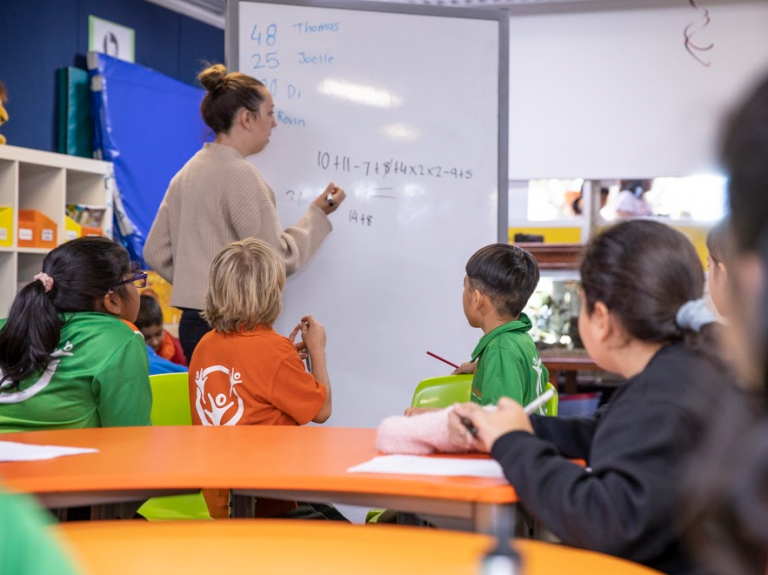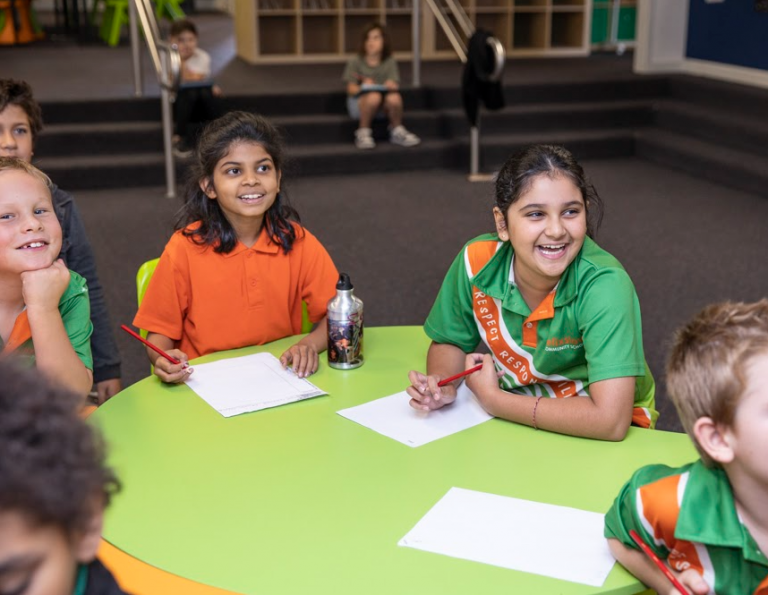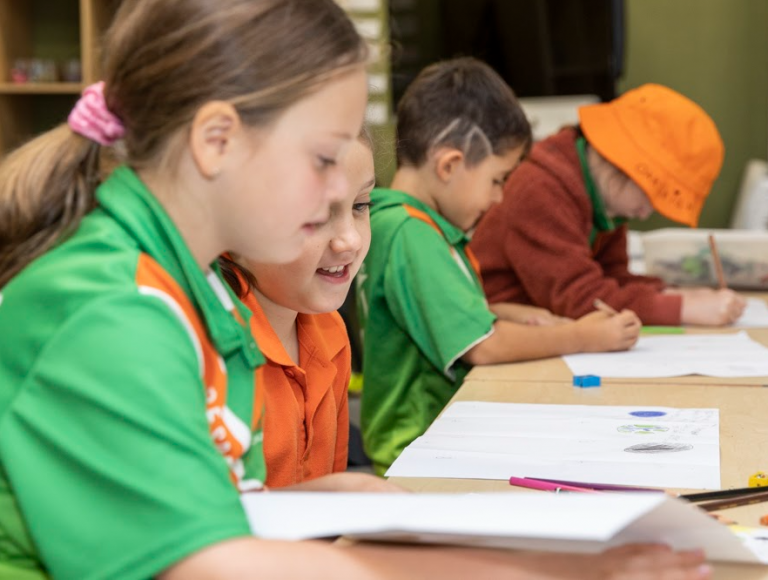# FelixstowPRIMARY SCHOOL# Numeracy

Numeracy is an area of the curriculum which requires explicit instruction. We engage in explicit Numeracy and Maths blocks four-five times per week to support students to know and understand the building blocks of mathematical concepts. Students also learn mathematical concepts through inquiry learning with a focus on problem solving and using mathematical thinking in context.

The planning and programming of Mathematics is designed to give students opportunities to:

• Develop strong numeracy skills in all areas of the ‘Big Ideas in Number’
• Choose and use mathematics
• Allow for the development of the proficiencies: understanding, fluency, problem solving, reasoning
• Develop positive dispositions and transferable skills for powerful learning
• Use mathematics in real life situationsWe work with the  ‘Big Ideas in Number’ guidelines to support students to develop strong number sense and skills.

### BIG IDEAS IN NUMBER:

1. Trusting the Count:  developing flexible mental objects for the numbers 0 to 10.
2. Place-value: the importance of moving beyond counting by ones, the structure of the base 10 numeration system.
3. Multiplicative thinking: the key to understanding rational number and developing efficient mental and written computation strategies in later years.
4. Partitioning: the missing link in building common fraction and decimal knowledge and confidence.
5. Proportional reasoning: extending what is known about multiplication and division beyond rule-based procedures to solve problems involving fractions, decimals, percent, ratio, rate and proportion.
6. Generalising: skills and strategies to support equivalence, recognition of number properties and patterns, and the use of algebraic text without which it is impossible to engage with broader curricula expectations at this level.